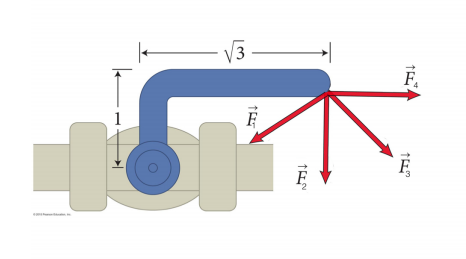# Problem: You want to rotate the L-shaped handle on a spigot (shown below) clockwise by pulling down on the end of the handle. Of the cases below, which force produces the largest magnitude torque on the spigot? (The magnitude of all the forces shown are the same.)(a) F1 (b) F2 (c) F3 (d) F4

###### FREE Expert Solution

Torque:

$\overline{){\mathbf{\tau }}{\mathbf{=}}{\mathbf{r}}{\mathbf{F}}{\mathbf{s}}{\mathbf{i}}{\mathbf{n}}{\mathbf{\theta }}}$ where r is the distance between F and the turning point, F is the force, and θ is the angle between r and F.

97% (494 ratings)###### Problem Details

You want to rotate the L-shaped handle on a spigot (shown below) clockwise by pulling down on the end of the handle. Of the cases below, which force produces the largest magnitude torque on the spigot? (The magnitude of all the forces shown are the same.)(a) F1 (b) F2 (c) F3 (d) F4